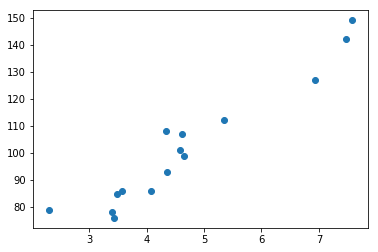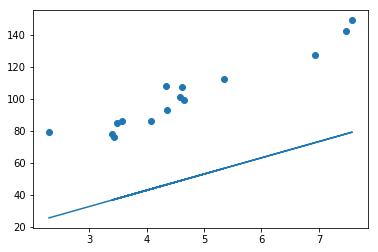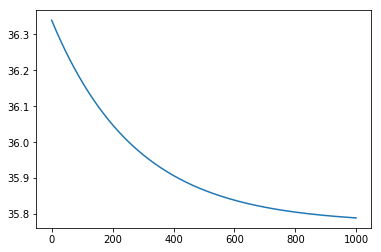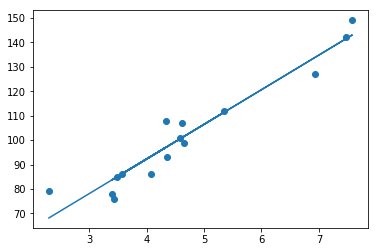# How much for my next Uber? Light intro to machine learning

Ok, lets break down the problem - we want to train a model to be able to predict price for an Uber based on my own driving history. It is important to realize that this problem is not just simple equation - where you could put multiple the kilometers by some fixed rate and have the price instantly. This problem depends on more variables than just distance - for example on the traffic and the waiting times, on the route which the driver chose, etc. Our goal is to let the computer to learn those nuances and predict the price without us telling it the details like base rate or rate per kilometers..

All we need is some existing data, so that the program can learn from it. If you want to follow the same example, you can create a simple dataset from your Uber account as I did - I just wrote an excel table with kilometers and prices from my history. You do not need to have hundreds of rows, I did just 15. The more, the more accurate the result, but since we are only considering one variable (kilometers), the model is so easy that 15 entries should be enough.

You can find the code with all the graphs and comments by the end of the article, so I will now go through the theory. Just a few practical notes: The code is written in Python, using few helpful libraries like NumPy or MatPlotLib. However, we are not going to use a machine learning library (as for example Tensorflow), because we want to learn the basic concepts. However I suggest to check it out at some point. I am also using Jupyter Notebook to write and run the code in the browser.

What is machine learning? As oppose to traditional algorithms, where you tell the computer what to do, machine learning is based on learning. The computer itself is progressively getting to better and better results, without a programmer telling him how to do it. There are several ways how to do machine learning and we will look at the category called supervised learning. It means that we will supervise the learning process - we will evaluate how the program is doing by comparing our results with the correct results. It also means we need to have the correct results available (they are called labels). Example would be getting a program to tell if there is a dog or a cat on the picture. During the learning process, we would give to the program some images, for which we would know the correct answer, and we would let the program to guess. We would then compare its guess to the correct answer and reward it accordingly.

In our Uber example we will do something similar - we will train our model based on existing data from past journeys and then we will ask about predictions for the future. We will use method called linear regression. We will try to find a line, which would describe the relationship between the kilometers and prices. We will put the line into the graph of those 2 variables, see the code bellow to understand this part.

We will start with something very silly - a horizontal line, which obviously does not describe our data at all. But how do we know if it represents our data or not? We need a method to tell - to estimate how bad it is. In machine learning, this is called cost function. There are plenty of ways how to evaluate the correctness, but since we know we want the line to fit the points (which are almost linear, as the dependence of price on kilometers is very strong), we want it to be close to all of the points. Hence we can use method called Mean squared error. It is computed like this:

\begin{align} cost &= \frac{1}{N} \sum_{i=1}^N (y_i - (m \cdot x_i))^2 \\ \end{align}

Now we can tell how much is the line off. What next? Now we want to minimize this number, as we want the error - the distance of the points from the line - to be the smallest. And here comes very important function for machine learning - gradient descent. Gradient descent is an algorithm to find a local minimum of a function. It is based on a gradient - which is a vector of all the partial derrivatives for all the function parameters. Those derivates tells us how the function would change if we would change the parameter. We can use this knowledge to change the parameters so that the function output is smaller than it was. This is exactly what gradient descent is doing - it is trying to get to the minimum of the function by iteratively changing its parameters so that the value of the function decreases.

We have 2 variables - or weights - and those are m and b, because the equation for a line is

$$y = m \cdot x + b$$

x and y are points from our datasets and m and b are wights which we will update in order to get to the minimum of the cost function. We want line which will have the minimum error - will be closest to our data.

Now we just need to calculate the gradients for m and b, and then update those values and try again - we will iterate this approach as long as we do not get to the minimum.

How to calculate the gradient? We need a little bit of calculus now because it is a partial derivative of our cost function with respects to m and b.

\begin{align} cost &= \frac{1}{N} \sum_{i=1}^N (y_i - (m \cdot x_i))^2 \\ \\ \frac{\partial cost}{\partial m} &= \frac{1}{N} \sum_{i=1}^N 2 \cdot (y_i - (m \cdot x_i + b)) \cdot (-x) \\ &= - \frac{2}{N} \sum_{i=1}^N x \cdot (y_i - (m \cdot x_i + b)) \\ \\ \frac{\partial cost}{\partial b} &= \frac{1}{N} \sum_{i=1}^N 2(y_i - (m \cdot x_i + b)) \cdot (-1) \\ &= - \frac{2}{N} \sum_{i=1}^N y_i - (m \cdot x_i + b) \\ \end{align}

Ok, now we have it almost done. The last step is to use this calculated gradients and multiply them with the original m and b. We will add one last thing - the learning rate, which will slow down our algorithm. You can learn more about it for example here.

And thats it, after few dozens of iterations we will get to the perfect line which we can use to predict the price based on kilometers. Here is the code with comments to go through it again, but this time programatically:

In :
# Importing libraries
%matplotlib inline
import numpy as np
import matplotlib.pyplot as plt


In :
# In this example we will try to predict price for an Uber based on kilometers
# load the data from the file - this dataset is a small personal dataset which I wrote based on my Uber account
data = np.genfromtxt("dataset_uber.csv", delimiter=",")
# this is how our data looks like - first column is price, second column kilometers and last column minutes
data


Out:
array([[ 107.  ,    4.62,   12.  ],
[ 112.  ,    5.34,   11.  ],
[ 101.  ,    4.59,   10.  ],
[  86.  ,    3.57,    8.  ],
[ 108.  ,    4.33,   13.  ],
[  76.  ,    3.43,    6.  ],
[  93.  ,    4.35,    8.  ],
[  78.  ,    3.4 ,    7.  ],
[ 127.  ,    6.94,   11.  ],
[ 149.  ,    7.58,   16.  ],
[ 142.  ,    7.47,   14.  ],
[  85.  ,    3.48,    9.  ],
[  86.  ,    4.07,    7.  ],
[  99.  ,    4.65,    9.  ],
[  79.  ,    2.3 ,   10.  ]])

In :
# X are our features - we will only care about kilometers to keep it simple
X = data[:,1]
# y are our labels - the correct answers, the actual prices
# we will use it to train our model - we are doing supervised learning
y = data[:,0]


In :
# Lets visualize our data - the price dependent on the kilometers
from mpltoolkits.mplot3d import Axes3D
fig = plt.figure()
ax.scatter(X, y)

plt.show()In :
# our weights will be parameters for a line
# since we will try to put the line through the data to make predictions
# line = m * x + b
m = 0
b = 0


In :
# our cost function will tell us how bad our line is - the closer to the points, to better
def cost(m, b, X, y):
ypred = m * X + b
err = ypred - y
return np.mean(err * err)


In :
# we will calculate gradient descent for both parameters
# it will tell us how to change the line to minimaze cost function
# it uses derrivate of our cost function with respects to m and b
gradientm = 2  np.mean(-X  (y - (m*X + b)))
gradientb = 2  np.mean(-1  (y - (m*X + b)))


In :
# lets call the grad function to get the gradients
dm, db = grad(m, b, X, y)


In :
# lets update our line parameters based on gradients and learning rate = 0.01
m = m - dm  0.01
b = b - db  0.01


In :
# lets see the new cost - how far are we from ideal line (close to all of the points)
cost(m,b,X,y)


Out:
2809.3022963954782

In :
# we can visualize how the line looks like now: we can see it is still far away
plt.scatter(X, y)
plt.plot(X, m * X + b)


Out:
[<matplotlib.lines.Line2D at 0x1146c1780>]In :
# Lets do the same steps as above but in a loop
# and lets keep track of our costs so that we can plot them
costs = []
for i in range(1000):
dm, db = grad(m, b, X, y)
m = m - dm  0.01
b = b - db  0.01
costs.append(cost(m,b,X,y))


In :
# we can see that the cost started as a high number but got into its minimum
plt.plot(range(1000), costs)


Out:
[<matplotlib.lines.Line2D at 0x1144027f0>]In :
# if we plot our new line now, we can see it fits perfectly the data
plt.scatter(X, y)
plt.plot(X, m * X + b)


Out:
[<matplotlib.lines.Line2D at 0x11430cf60>]In :
# Our model is DONE! We can make predictions like:
# how much will it cost to drive 5 km?
def predict(km):
return m * km + b

predict(5)


Out:
106.44071995372065

In [ ]:
# We can see it will cost aprox. 106 Kc.
# And thats it, we trained our model based on training data and optimized it via gradient descent!